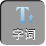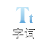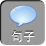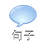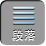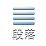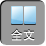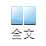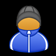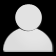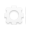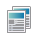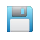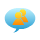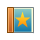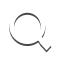-AA+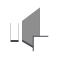1、单项式：由数与字母或字母相乘组成的代数式叫做单项式，单独的一个数或一个字母也是单项式，单项式的系数：单项式中的数字因数叫做单项式的系数.

2、多项式：由几个单项式相加组成的代数式叫做多项式，其中每个单项式叫做多项式的项.多项式的次数：多项式里，次数最高的项的次数就叫做这个多项式的次数，其中不含字母的项叫做常数项

1、把下列代数式的代号填在相应的横线上：① a 2 b+ ab 2;②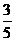x-x 2+ 1;③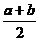;④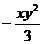;⑤ 0;⑥ - x+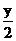;⑦ a 2+ ab 2+ b 3;⑧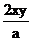;⑨ 3 x 2+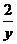.

( 1)单项式：________________________；

( 2)多项式：________________________;

( 3)整式:___________________________;

( 4)三次多项式：_____________________；

( 5)非整式：__________________________.

2、指出多项式 2 x 3 y -4 y 2+ 5 x 2的项、次数、并说明它是几次几项式，然后把它按 x的降幂排列、按 y的升幂排列.

x的降幂排列： 2 x 3 y+ 5 x 2 -4 y 2；按 y的升幂排列： -4 y 2+ 2 x 3 y+ 5 x 2.

3、观察下列一串单项式的特点： xy ,-2 x 2 y ,4 x 3 y ,-8 x 4 y ,16 x 5 y,...

( 1)按此规律写出第 9个单项式；

( 2)试猜想第 n个单项式为多少？它们的系数和次数分别是多少？

2）根据单项式的系数是指单项式的数字因数，次数是所有字母指数的和解答即可。

2）第 n个单项式为（ -1 n+ 1 2 n -1,次数： n+ 1.

4、已知多项式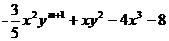是七次多项式，单项式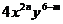与该多项式的次数相同，试求 mn的值.

5、已知代数式 ax 5+ bx 3+ 3 x+ c,当 x= 0时，该式的值为 -1.

1）求 c的值；

2）已知当 x= 1时，该式的值为 -1，试求 a+ b+ c的值；

3）已知当 x= 3时，该式的值为 -10，试求当 x= -3时该式的值；

4）在第（ 3）题的已知条件下，若有 3 a= 5 b成立，试比较 a+ bc的大小.

2）将 x= 1代入代数式即可求出 a+ b+ c的值；

3）将 x= 3代入代数式求出代数式的值，再将 x= -3代入代数式，变形后即可；

4）与（ 3）的方法相同；

2）把 x= 1代入代数式 ax 5+ bx 3+ 3 x+ c，得到 a+ b+ 3+ c= -1,所以 a+ b+ c= -4;

3）把 x= 3代入代数式 ax 5+ bx 3+ 3 x+ c，得到 3 5 a+ 3 3 b+ 9+ c= -10,即 3 5 a+ 3 3 b= -18.

x= -3时，原式= -3 5 a -3 3 b -9-1= 8.

4）由（ 3）得到 3 5 a+ 3 3 b= -18，即 27 a+ 3 b= -2.

6、观察下列等式：

1个等式： a 1=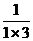=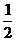×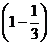;

2个等式： a 2=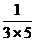=×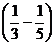;

3个等式： a 3=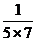=×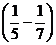;

4个等式： a 4=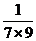=×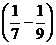;

1）按以上规律列出第 5个等式:__________________；

2）用含有 n的代数式表示第 n个等式：___________________；

3）求 a 1+ a 2+ a 3+ a 4++ a 100的值

( 2) a n=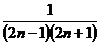=×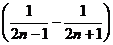;

( 3) a 1+ a 2+ a 3+ a 4++ a 100=×+×+×++×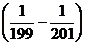=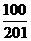.

1、已知代数式 x 2+ x+ 1的值是 8，那么代数式 4 x 2+ 4 x+ 9的值是__________；

2、如果多项式 4 x 4+ 4 x 2-3 x n+ 2+ 5 x的次数相同，求代数式 3 n -4的值.

137

2n= 4,则代数式的值为： 8.